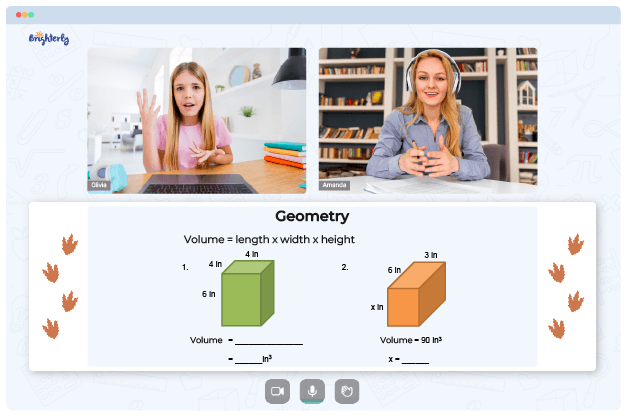# Lines of Symmetry Worksheets

The knowledge on lines of symmetry is essential in studying math, especially in geometry, because it helps students understand the properties and characteristics of various shapes. Students can use a line of symmetry worksheet to practice and put their knowledge of this idea into practice. As you go through this article, you’ll understand what lines of symmetry worksheets are.

A line of symmetry is a line that divides an object or shape into two identical parts that are mirror images of each other. Vertical, horizontal, and diagonal lines are the most prevalent types of lines of symmetry.

Math for Kids

Is Your Child Struggling With Math?
1:1 Online Math TutoringFor vertical lines, the shape is divided into two equal parts by a line of symmetry that runs from top to bottom, with one part being a mirror image of the other. For horizontal lines, the shape is divided into two equal parts by a line of symmetry that runs from left to right. For diagonal ones, the shape is divided into two equal parts by a diagonal line of symmetry that runs at an angle and divides one part into a mirror image of the other.

With lines of symmetry 4th grade worksheet, a shape is divided into two identical halves. Students can identify symmetrical shapes and draw lines of symmetry with the assistance of symmetry worksheets. Your child can practice recognizing and drawing lines of symmetry on shapes and symbols using the lines of symmetry worksheets. And they aren’t just for fourth graders. Symmetry worksheets work for kids in grades 3 through 5, helping them comprehend the lines of symmetry in multiple shapes.

## Benefits of Lines of Symmetry Worksheets

Students can practice and use their knowledge of lines of symmetry with the help of drawing lines of symmetry worksheets. The tool helps students identify rotational symmetry and recognize lines of symmetry in various letters and shapes. For instance, students might be asked to draw a shape with two symmetrical lines or a letter with three lines of symmetry. They can also learn to use rotational symmetry to get out of tight spots and solve distribution problems.### Lines of Symmetry Worksheets PDF

Line Of Symmetry Worksheet### Lines of Symmetry Worksheets PDF

Lines Of Symmetry 4Th Grade Worksheet### Lines of Symmetry Worksheets PDF

Lines Of Symmetry Worksheet 4Th Grade### Lines of Symmetry Worksheets PDF

Lines Of Symmetry Worksheet

Struggling with Geometry?• Does your child struggle with understanding the basics of geometry?
• Try studying with an online tutor.

Is your child having trouble mastering the concept of geometry? An online tutor could provide the necessary guidance.